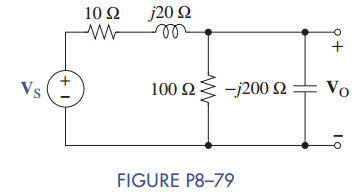Chat Now

# Find the phasor input V S in Figure P8–79 when the phasor output is V O = 300 + j200 V.

### Find the phasor input V S in Figure P8–79 when the phasor output is V O = 300 + j200 V.

Find the phasor input VS in Figure P8–79 when the phasor output is VO = 300 + j200 V.manish jayant 27-Jul-2020Get solution